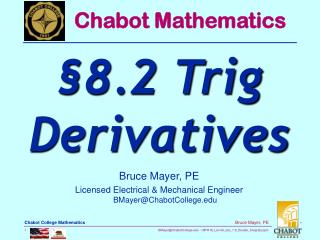# Bruce Mayer, PE Licensed Electrical & Mechanical Engineer BMayer@ChabotCollege - PowerPoint PPT PresentationDownload PresentationBruce Mayer, PE Licensed Electrical & Mechanical Engineer BMayer@ChabotCollege

Download Presentation## Bruce Mayer, PE Licensed Electrical & Mechanical Engineer BMayer@ChabotCollege

- - - - - - - - - - - - - - - - - - - - - - - - - - - E N D - - - - - - - - - - - - - - - - - - - - - - - - - - -
##### Presentation Transcript

1. Chabot Mathematics §8.2 TrigDerivatives Bruce Mayer, PE Licensed Electrical & Mechanical EngineerBMayer@ChabotCollege.edu

2. 8.1 Review § • Any QUESTIONS About • §8.1 → TrigonometricFunctions • Any QUESTIONS About HomeWork • §8.1 → HW-10

3. §8.2 Learning Goals • Derive and use differentiation formulas for trigonometric functions • Study periodic rate and optimization problems using derivatives of trigonometric functions

4. Derivatives for Sine and Cosine • For independent variable t measured in Radians • Use the ChainRule when the sin/cos arguments are a function of t, u(t)

5. Verify Trig Derivs • Use SpreadSheet to Check that

6. Trig Deriv Proof • Prove: • Recall DerivateDefinition • Use the TrigSum-Identity • Apply TrigID to Limit

7. Trig Deriv Proof • Factor the Limit argument • By Limit Properties (c.f. §1.5) • Now Two Limits whose Proof is Beyond the Scope of MTH16:

8. Trig Deriv Proof • Using these Limits • Then Finally Q.E.D.

9. Example  CoSine Derivative • Find: • SOLUTION: Use the Product Rule

10. Example  Maximizing Microbes • An approximate Math Model for the population of microbes present at temperature T: • Where • T in Degrees Celsius (°C) • P in Millions of Microbes (MegaMicrobes, MM) • What is the population when the microbial population is decreasing most rapidly?

11. Example  Maximizing Microbes • SOLUTION: • The population Decreases most rapidly when the derivative of the population function; i.e. the GrowthRatedP/dt, is minimized. • to minimize the first derivative, find the critical points, which requires computation of the 2nd derivative and 2nd derivative zeroes.

12. Example  Maximizing Microbes • Taking the Derivatives

13. Example  Maximizing Microbes • Set to Zero the 2nd Derivative • The above eqn has infinitely many solutions, but recall that the T-domain Restriction: [0,50].

14. Example  Maximizing Microbes • The simplest solutions to sin(θ)=0 are 0 and π. However, any solution that is a multiple of 2π away from either solution is also a solution. Thus • Where k is any Integer • Solving for T find

15. Example  Maximizing Microbes • Then the two branches of solutions in terms of T: • The only solutions for T on the interval [0,50] are 0 and 30 • Need to consider both critical points, as well as the endpoints 0 (0 is also a critical point) and 50, then note which input corresponds to the smallest (most negative) value of dP/dT

16. Example  Maximizing Microbes • Tabulating the Results • The only negative dP/dT is at T = 30, which then corresponds to the minimum • Then the microbial population at 30 °C:

17. Derivatives for tan and sec • For independent variable t measured in Radians • Use the ChainRule when the tan/sec arguments are a function of t, u(t)

18. tan Trig Deriv Proof • Prove: • Use the • Tan definition: • Quotient Rule: • Previously Proved Trig Derivs: • Then

19. tan Trig Deriv Proof • Using the Quotient Rule and Chain Rule • Or • Using another Trig ID → • Find

20. Example  tan derivative • Find

21. Example  Related Rate & trig • A birdwatcher observes a bird flying overhead away from her. She estimates that the bird is flying parallel to the ground at 10 mph and is initially 40 feet away horizontally and 15 feet above the birdwatcher’s line of sight. • How quickly is the angle between the birdwatcher’s light of sight and the location of the bird changing after 12 seconds?

22. Example  Related Rate & trig • A diagram REALLY helps in this case. • Let • W ≡ the initial location of the birdwatcher • B ≡ the current position of the bird • ThentheDiagram:

23. Example  Related Rate & trig • SOLUTION: • First find the rate of change in θ with respect to time. The relationship between the angle and the given distances can be represented by the tangent function (opposite over adjacent)

24. Example  Related Rate & trig • Use implicit differentiation to take derivatives of both sides with respect to time, noting that h is constant:

25. Example  Related Rate & trig • To find dθ/dt replace all of the other variables with their values at the time when the bird has been flying for 12 seconds. • First, the value of x is initially 40 ft, but after 12 seconds flying at 10 mph, the horizontal distance increases to

26. Example  Related Rate & trig • Use x = 216ft after to find sec2θ after the 12 second Flite Time: • Now use the Pythagorean identity relating tan and sec • Thus

27. Example  Related Rate & Trig • Now combine all of the values into the implicit differentiation equation: • After 15 seconds, the angle of inclination to the bird decreases at about 1.59 degrees per second.

28. WhiteBoard Work • Problems From §8.2 • P8.2-50 → RowBoat Rope Reel-In • P8.2-57 →HarmonicMotion

29. All Done for Today Trig DerivChainRule

30. Chabot Mathematics Appendix Do On Wht/BlkBorad Bruce Mayer, PE Licensed Electrical & Mechanical EngineerBMayer@ChabotCollege.edu –